As was shown in the last chapter with systems of equations, linear transformations can also be written in matrix form. If we take the linear function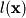from the previous chapter as an example (we'll call it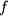here):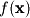: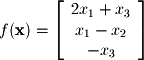we can write this function as the multiplication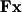: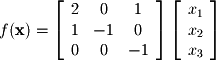It is easy to verify thatis equivalent tothrough matrix multiplication. Thus, multiplying any matrix by a vector is equivalent to performing a linear transformation on that vector. Thus, the matrix form is a very convenient way of representing linear functions.

In addition to multiplying a transform matrix by a vector, matrices can be multiplied in order to carry out a function convolution. Consider another linear function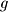: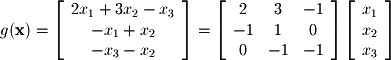If we convolve the two functions, it is easy to show (although it will not be done here) that the convolution is equivalent to the following: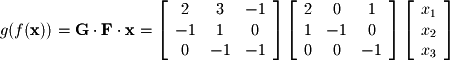Keep in mind that since matrix multiplication is not commutative that the order of the multiplication is important. The grouping of the multiplication is not important since matrix multiplication is associative. In other words, you can perform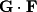first or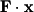first and still get the same result. By doing the former you are essentially transforming the transform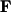first and then transforming the vector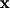. In the latter you are transforming the vectorbyand then passing it to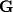to be transformed again. These two operations are equivalent, but switching the multiplication so thatis transformed bybeforedoes not yield an equivalent result.